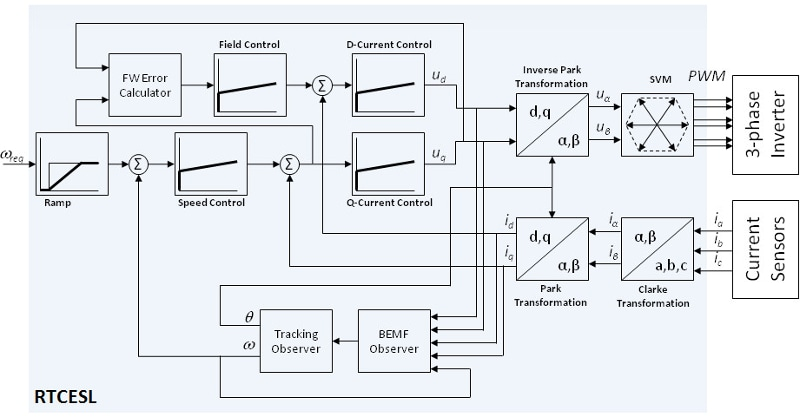RTCESL: Real Time Control Embedded Software Motor Control and Power Conversion Libraries

Embedded Software and Motor Control Libraries Block DiagramTarget Applications

Implemented Algorithms in RTCESL 4.4

Algorithm (16 and 32-bit fixed point, 32-bit floating point) Core Supported
DSP56800E DSP56800EX Cortex M7 Cortex M4 Cortex M0+
Absolute value x x x x x
Negation x x x x x
Conversion x x x x x
Conversion with rounding x x x x x
Addition x x x x x
Leading-bit count x x x x x
Subtraction x x x x x
Single-bit Shift x x x x x
Multi-bit Shift x x x x x
Mutli-bib bidirectional shift x x x x x
Mutliplication x x x x x
Mutliplication with negation x x x x x
Mutliplication with rounding x x x x x
Mutliplication with negation and rounding x x x x x
Multiplication-accumulation x x x x x
Multiplication-negation-accumulation x x x
Multiplication-subtraction x x x x x
Multiplication-negation-accumulation with rounding x x x
Multiplication-accumulation with rounding x x x x x
Multiplication-subtraction with rounding x x x x x
Division (single quadrant) x x x x x
Signed division x x x x x
Reciprocal (single-quadrant) x x x x x
Signed reciprocal x x x x x
Sign x x x x x
Binary logarithm x x x x x
Saturation x x x x x
Sum of four addends x x x x x
Subtraction of 3 subtrahends from the minuend x x x x x
Addition of two products of two multiplicands x x x x x
Addition of two products of two multiplicands with rounding x x x x x
Subtraction of a product of last two multiplicands from the product of first two multiplicands x x x x x
Subtraction of a product of last two multiplicands from the product of first two multiplicands with rounding x x x x x
Algorithm (16 and 32-bit fixed point, 32-bit floating point) Core Supported
DSP56800E DSP56800EX Cortex M7 Cortex M4 Cortex M0+
Sine x x x x x
Cosine x x x x x
Tangent x x x x
Arcus Sine x x x x
Arcus Cosine x x x x
Arcus Tangent x x x x x
Arcus Tangent YX x x x x x
Square Root x x x x x
Limitation x x x x x
Lower Limitation x x x x x
Upper Limitation x x x x x
Vector Limitation x x x x
Vector Limitation 1 x x x x x
Hysteresis x x x x x
Look-up table 1D x x x x x
Periodical Look-up table 1D x x x x x
Look-up table 1D (32 bit) x x x
Periodical look-up table 1D (32 bit) x x x
Ramp x x x x x
Dynamic Ramp x x x x x
Flex Ramp x x x x x
Dynamic Flex Ramp x x x x x
Integrator x x x x x
Flex S Ramp x x
Parallel form of PI controller with anti-wind-up x x x x x
Parallel form of PID controller with anti-wind-up x x x x
Parallel form of Beta IP controller with anti-wind-up x x x
Parallel form of Beta IPD controller with anti-wind-up x x
Algorithm (16 and 32-bit fixed point, 32-bit floating point) Core Supported
DSP56800E DSP56800EX Cortex M7 Cortex M4 Cortex M0+
Clarke Transformation x x x x x
Inverse Clarke Transformation x x x x x
Park Transformation x x x x x
Inverse Park Transformation x x x x x
PMSM Decoupling x x x x x
DC Bus Ripple Elimination for FOC x x x x x
DC Bus Ripple Elimination x x x x x
Space Vector Modulation-standard x x x x x
Space Vector Modulation-with 0 000 nulls x x x x x
Space Vector Modulation-with 0 111 nulls x x x x x
Space Vector Modulation-inverse Clarke Transformation x x x x x
Standard Discontinuous PWM x x x
Extended Discontinuous PWM x x x
Algorithm (16 and 32-bit fixed point, 32-bit floating point) Core Supported
DSP56800E DSP56800EX Cortex M7 Cortex M4 Cortex M0+
1st Order IIR Filter x x x x x
2nd Order IIR Filter x x x x x
3rd Order IIR Filter x x x x
4th Order IIR Filter x x x x
Moving Average Filter x x x x x
Exponential filter x x x
Algorithm (16 and 32-bit fixed point, 32-bit floating point) Core Supported
DSP56800E DSP56800EX Cortex M7 Cortex M4 Cortex M0+
Tracking Observer x x x x x
Angle Tracking Observer x x x x x
PMSM BEMF Observer in D/Q x x x x x
PMSM BEMF observer in A/B x x x
ACIM rotor flux observer x x
ACIM speed MRAS estimator x x
ACIM MTPA controller x x
Algorithm (16 and 32-bit fixed point, 32-bit floating point) Core Supported
DSP56800E DSP56800EX Cortex M7 Cortex M4 Cortex M0+
PI controller x x x x x
PI with low-pass filter controller x x x x x
PID controller x x x x x
2P-2Z controller x x x x x
3P-3Z controller x x x x x